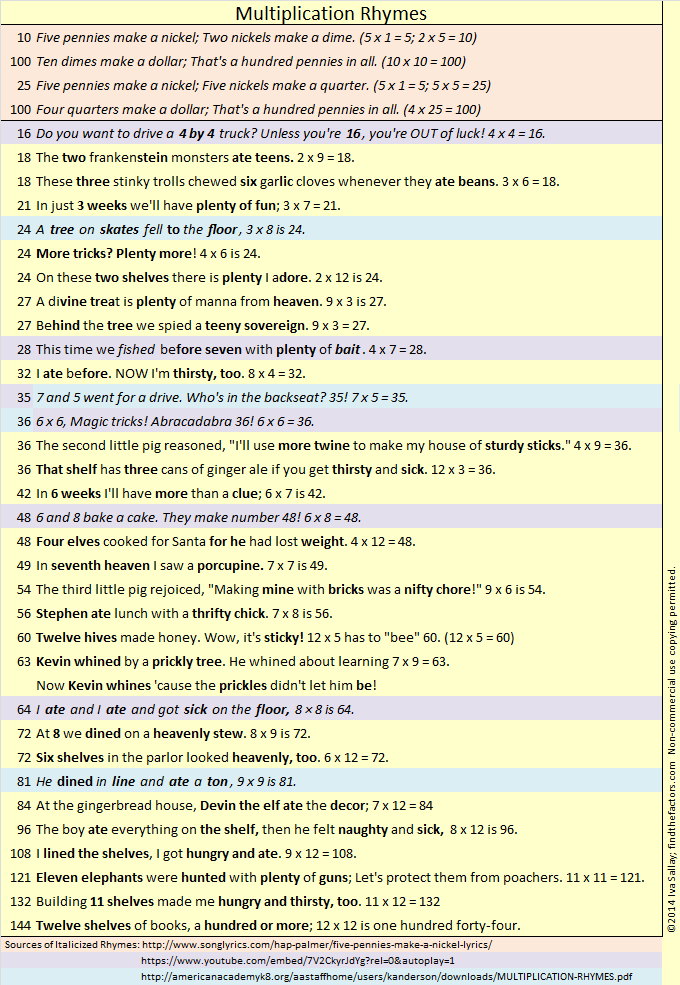# 1193 Math Carnival Games

During the last week of every month, there is a math education blog carnival happening somewhere in the blogosphere. This month it will happen on my blog! Why do I get to host it? I sent an email to Denise Gaskins who coordinates the carnival and requested the privilege. If you would like to host it in the future, let her know.

In the meantime, you can help me with my carnival. Math can be so much fun for kids from preschool age and even all the way up to high school. If you blog about that, I would love to include one or more of your posts in my carnival. You’ve poured your heart and soul into your posts, so why not promote it at no cost to you?  Don’t be shy! I want to read it, and other people will want to read it, too.

The deadline for submitting posts to my carnival is Friday, August 24th. There is a form for you to submit a link to your post on Denise Gaskins website. Then the following week you will be able to enjoy the carnival even more because of your participation!Now it will be my pleasure to tell you a few facts about the number 1193:

• 1193 is a prime number.
• Prime factorization: 1193 is prime.
• The exponent of prime number 1193 is 1. Adding 1 to that exponent we get (1 + 1) = 2. Therefore 1193 has exactly 2 factors.
• Factors of 1193: 1, 1193
• Factor pairs: 1193 = 1 × 1193
• 1193 has no square factors that allow its square root to be simplified. √1193 ≈ 34.53983

How do we know that 1193 is a prime number? If 1193 were not a prime number, then it would be divisible by at least one prime number less than or equal to √1193 ≈ 34.5. Since 1193 cannot be divided evenly by 2, 3, 5, 7, 11, 13, 17, 19, 23, 29 or 31, we know that 1193 is a prime number.1193 is the sum of five consecutive prime numbers:
229 + 233 + 239 + 241 + 251 = 1193

32² + 13² = 1193

1193 is the hypotenuse of a Pythagorean triple:
832-855-1193 calculated from 2(32)(13), 32² – 13², 32² + 13²

Here’s another way we know that 1193 is a prime number: Since its last two digits divided by 4 leave a remainder of 1, and 32² + 13² = 1193 with 32 and 13 having no common prime factors, 1193 will be prime unless it is divisible by a prime number Pythagorean triple hypotenuse less than or equal to √1193 ≈ 34.5. Since 1193 is not divisible by 5, 13, 17, or 29, we know that 1193 is a prime number.

# 1192 Experiential Learning Helps Students Understand Their Capacity

Sara Van Der Werf inspired me last year with her post titled Why You Need a PLAY TABLE in your mathematics classroom ASAP. I’ve seen merit in using play to teach and learn mathematics for some time now. What Sara’s post did was make me long to be back in a mathematics classroom so I could have such a play table!

Last week I started working at the American Academy of Innovation, a charter school nearby that engages students in learning through projects and other activities.

Each member of the faculty was given the assignment to make something that reflects his or her teaching philosophy. Here’s the one I put together:On my display, I included the words, “Experiential Learning Helps Students Understand Their Capacity.” That’s my teaching philosophy.

The wording I used was intentional.

•  “Their” might refer to the students or to the geometric solids.
• “Capacity” has two appropriate definitions stated so perfectly by Google in the screenshot below:
1) the ability or power the students “have to do, experience, or understand something”, and 2) the maximum amount the geometric solids can contain.
The scattered rice symbolizes that these learning experiences can sometimes be messy. We will learn more if we aren’t afraid to make mistakes or a little mess.

I am so excited! I’ve taken all my math toys out of storage and will have an ever-changing play table in my classroom where students can play and learn mathematics. Thank you, Sara Van Der Werf for inspiring me!

Now I’ll write a little about the number 1192:

• 1192 is a composite number.
• Prime factorization: 1192 = 2 × 2 × 2 × 149, which can be written 1192 = 2³ × 149
• The exponents in the prime factorization are 3 and 1. Adding one to each and multiplying we get (3 + 1)(1 + 1) = 4 × 2 = 8. Therefore 1192 has exactly 8 factors.
• Factors of 1192: 1, 2, 4, 8, 149, 298, 596, 1192
• Factor pairs: 1192 = 1 × 1192, 2 × 596, 4 × 298, or 8 × 149
• Taking the factor pair with the largest square number factor, we get √1192 = (√4)(√298) = 2√298 ≈ 34.525351192 is the sum of two consecutive prime numbers:
593 + 599 = 1192

34² +  6² = 1192

1192 is the hypotenuse of a Pythagorean triple:
408-1120-1192 calculated from 2(34)( 6), 34² –  6², 34² +  6²
It is also 8 times (51-140-149)

1192 is a cool-looking 3322 in BASE 7

# 917 How to Solve for X with Candy

How many m&m’s are there in one fun size Halloween pack of m&m’s?

I don’t know. You don’t know. Nobody knows. That’s why these little fun size packs make the perfect UNKNOWN. For this activity, there may even be a negative number of m&m’s in a pack because I’m only using blue and orange m&m’s, and I’m letting each blue m&m equal negative one and each orange m&m equal positive one.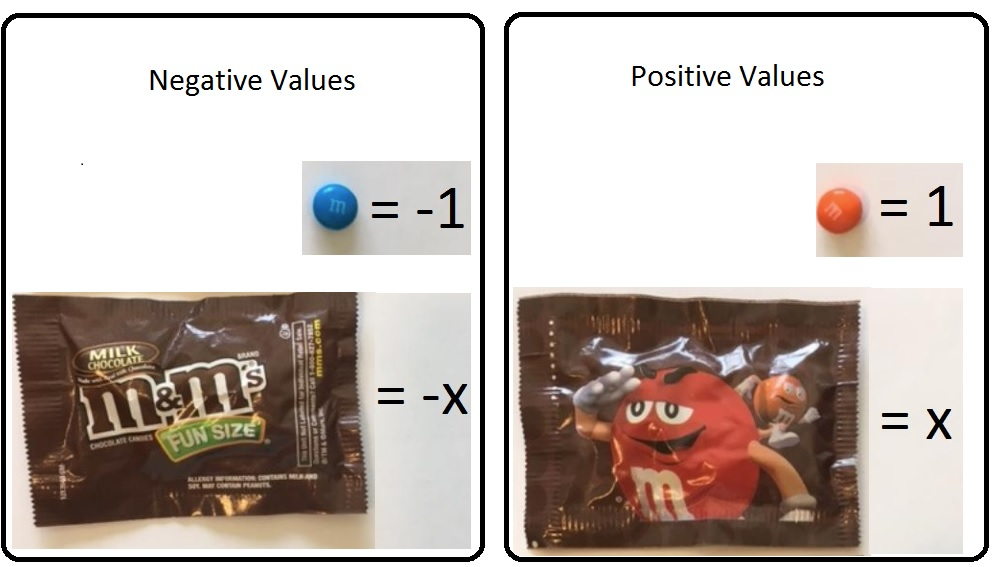I’m also letting the front side of the fun size package equal negative x, and the back side equal positive x. In algebra we often call our unknown x.

(The colors chosen don’t matter, as long as there are only two of them, and you are consistent with that color being positive or negative. The front of the package could just as easily be +1 and the back -1. Consistency is important. Choose the values you want to use and stick with them. You can also use ALL the m&m’s in a few single packs and have the side of the candy with the m be positive and the side without the m be negative. You can use the empty wrappers as x and -x.)

We can figure out how many m&m’s are in the pack by balancing an equation. The number of m&m’s in a pack is x. We will solve for x by using the very best algebra tiles in the world, m&m’s!

Besides fun size m&m’s (or Skittles or Reece’s Pieces) we need a paper balance for our equations:Click Equation Balance for a printable pdf of the paper balance.

Now let’s solve x – 3 = 5 by using the m&m’s to find x. This is how the equation balance should look to begin:We want to get the wrapper by itself, so what do we do? To keep the equation balanced, we add three (positive) orange m&m’s (one at a time) to both sides of the paper balance:Three (negative) blue m&m’s plus three (positive) orange m&m’s are equal to zero, so we can remove them.Mmm. I just ate zero m&m’s, and they tasted so good! That leaves us with x = 8, so we have found x, and the equation has been solved! (If you have more equations to solve, you might want to wait to eat the m&m’s until you’re just about finished.)

Now let’s try finding x when the equation is a little more complicated, -2x = x + 12. This is how the balance should look at the beginning:We want to get all the x’s on one side so we subtract x from both sides of the equation by adding a (negative) front-facing wrapper to both sides of the balance.Since x – x = 0, we can remove the front-facing and back-facing wrappers from the right side of the equation: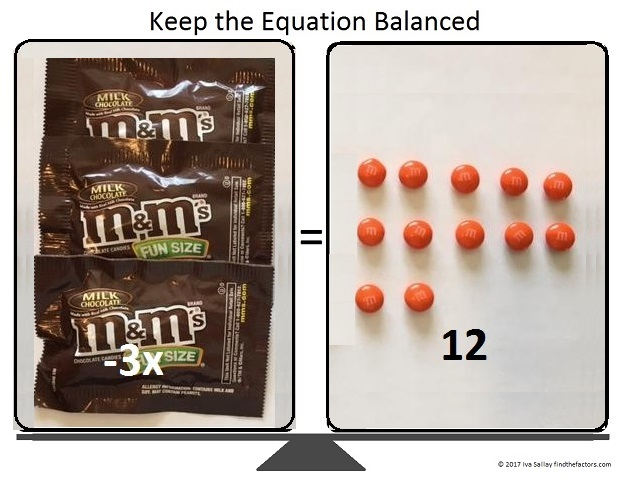We can arrange the 12 candies into 3 rows of 4.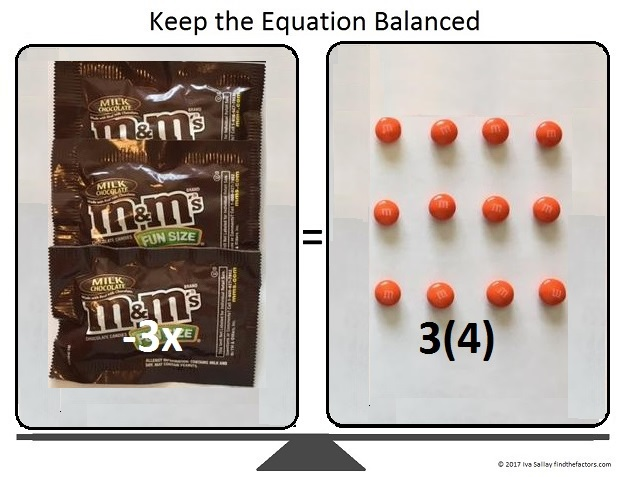Now we can divide both sides of the equation by 3.All that’s left to do is change the signs of EVERYTHING on both sides of the equation: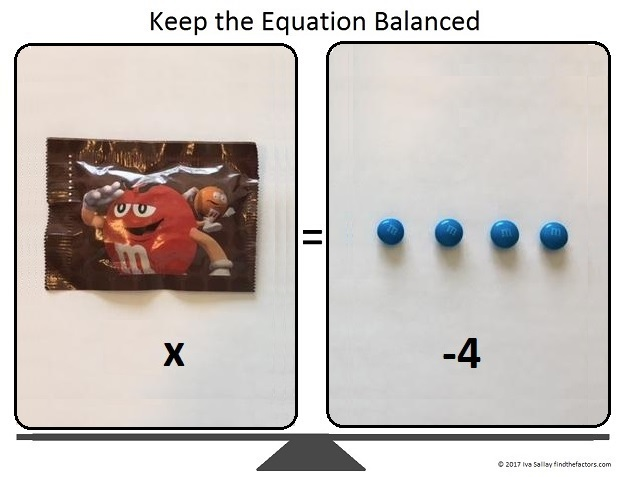Thus x = -4. We solved for x correctly because we kept the equation balanced every step of the way.

Now let me tell you a little bit about the number 917:

917 is the sum of five consecutive prime numbers:
173 + 179 + 181 + 191 + 193 = 917

Rearrange its digits and 917 becomes 197 in BASE 26.

• 917 is a composite number.
• Prime factorization: 917 = 7 × 131
• The exponents in the prime factorization are 1 and 1. Adding one to each and multiplying we get (1 + 1)(1 + 1) = 2 × 2 = 4. Therefore 917 has exactly 4 factors.
• Factors of 917: 1, 7, 131, 917
• Factor pairs: 917 = 1 × 917 or 7 × 131
• 917 has no square factors that allow its square root to be simplified. √917 ≈ 30.282007859# 884 Put First Things First

Tangrams have two large pieces, three medium size pieces, and two small pieces.Most Tangram puzzles are easier to solve if you figure out where to put the two big triangles first. Making a daily plan is easier if you figure out where to schedule the important items like homework and chores first. One of the Seven Habits of Highly Effective People is Put First Things First.

I wrote a 30-40 minute lesson plan to teach habit number 3 of the seven habits with the seven Tangram shapes. The lesson has now been taught to a third of a local elementary school. It was taught to students from first grade to sixth, and all of them really liked the lesson. The teachers who taught it enjoyed it very much as well. The rest of the school will be taught the same lesson later.

I made a pdf copy of the lesson plan here: Put First Things First. You can use it if you would like to teach that principle to your children or your students.  Part of the lesson is reading the adorable book, A Small Brown Dog with a Wet Pink Nose, by Stephanie Stuve-Bodeen. Our county library system had more than enough copies for us to use. It is also available on Amazon.com.The Tangram square above was copied on light brown paper so each student in the class could make their own small brown dog.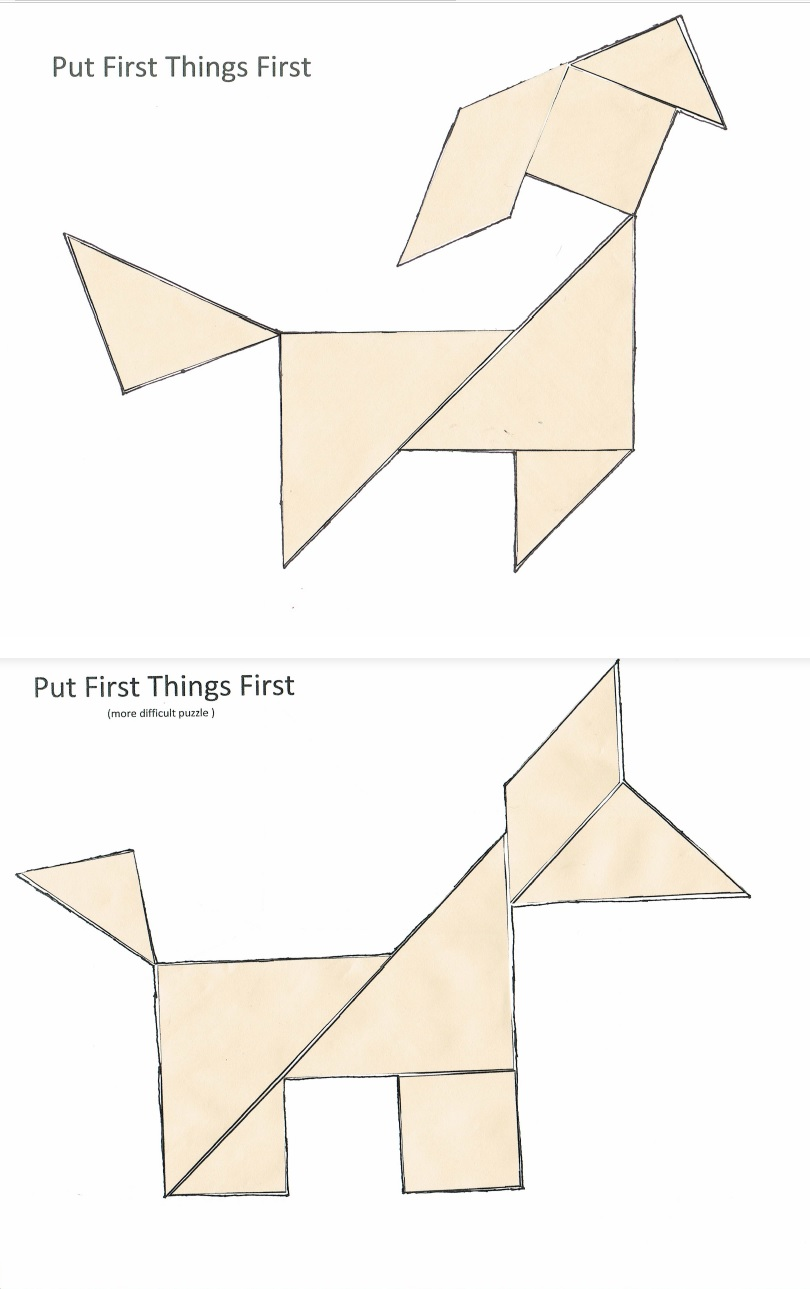Students could make both dogs or just one of them. Ability levels vary in surprising ways. There were a few first graders who could put the Tangram puzzle together without any help while a few of the older kids struggled. It was okay if a student had difficulty putting the puzzle together. In fact, I made that potential difficulty an important part of the lesson plan. After playing with the puzzle pieces, some students chose to glue the pieces onto the puzzle. Some of them used crayons to add details to their dogs.

The book and the puzzle were the funnest parts of the lesson, but the lesson began with a serious discussion. We used a document camera to show the four time quadrants to the classes.(I used the term “Pressing” instead of the more common term “Urgent.” Elementary students probably don’t know what either of those words mean, but they do know what “Pressure” means. They will feel a lot of pressure if they wait until the last minute to do something important. They might feel peer pressure to follow the crowd. Pressing and pressure have the same root word.)

So go ahead, click on the pdf file, Put First Things First, and teach planning and responsibility using the seven Tangram shapes.

Now I’ll write a little bit about the number 884:

884 is the hypotenuse of four Pythagorean triples.

• 84-880-884 which is 4 times (21-220-221)
• 340-816-884 which is (5-12-13) times 68
• 416-780-884 which is (8-15-17) times 52
• 560-684-884 which is 4 times (140-171-221)

If we had more than ten fingers, 884 might be written in some of these interesting ways:

• 2D2 in BASE 18 (D is 13 base 10), because 2(18²) + 13(18) + 2(1) = 884
• 202 in BASE 21, because 2(21²) + 2(1) = 2(441 + 1) = 2(442) = 884
• QQ in BASE 33 (Q is 26 base 10), because 26(33) + 26(1) = 26(34) = 884
• Q0 in BASE 34 because 26(34) = 884

What are the factors of 884?

• 884 is a composite number.
• Prime factorization: 884 = 2 × 2 × 3 × 73, which can be written 884 = 2² × 13 × 17
• The exponents in the prime factorization are 2, 1, and 1. Adding one to each and multiplying we get (2 + 1)(1 + 1)(1 + 1) = 3 × 2 × 2 = 12. Therefore 884 has exactly 12 factors.
• Factors of 884: 1, 2, 4, 13, 17, 26, 34, 52, 68, 221, 442, 884
• Factor pairs: 884 = 1 × 884, 2 × 442, 4 × 221, 13 × 68, 17 × 52, or 26 × 34,
• Taking the factor pair with the largest square number factor, we get √884 = (√4)(√221) = 2√221 ≈ 29.732137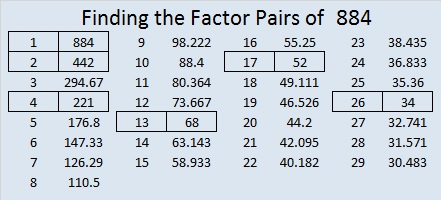884 is in this cool pattern: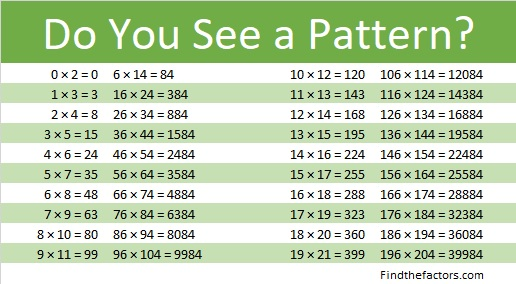# 111 and How Well Can You Add and Subtract in All Those Foreign Languages in Which You’ve Learned How to Count?

In general, adults understand how to add and subtract one-digit numbers, and most adults also have learned how to count in at least one language other than their native language. Does that mean these adults can add and subtract in those other languages in which they have learned how to count? I am curious to know the answer to that question. Let me tell you how counting, adding, and subtracting in a foreign language work for me.

I studied Spanish for three years in High School. I remember doing some basic addition and subtraction problems back then. Even though I remember very little Spanish vocabulary, I can still add and subtract one-digit numbers in Spanish fairly well.

I studied German for one and a half years in college. I remember even less German than I remember Spanish. All of the number words LOOK familiar, but I failed miserably when I tried to add and subtract using German numbers.

I lived in Turkey for two years when my husband was stationed at İncirlik Air Base near Adana. I learned a bit of vocabulary and how to count in Turkish. I actually remember the Turkish numbers a little better than the German ones, but my ability to add and subtract in Turkish isn’t much better.Now I am motivated to learn Hungarian. (I want to be able to understand my husband’s relatives better someday when I get to visit them in Hungary again.) The numbers in Hungarian are fresh in my mind, and I have even passed the time counting up to 1000 in Hungarian when I’ve gone for a walk.

When it came to doing basic addition or subtraction in Hungarian at this game site I found my ability to count did not help as much as I supposed. However, with PRACTICE, I have been able to greatly improve my ability to add and subtract in Hungarian.

After gaining the ability to add and subtract in Hungarian, I added another wrench to the process: I required myself to say complete number sentences in Hungarian instead of allowing English words like plus, minus, or equals to be used.From Hungarian Verbs & Essentials of Grammar, I learned that there are a few ways to say a number sentence when adding in Hungarian. The easiest two ways to say 4 + 3 = 7 are “Négy meg három (az) hét” or “Négy plusz három (az) hét.” Subtraction is a little more complicated because different numbers take two different case endings, ból or ből, in order to maintain vowel harmony. 10 – 4 = 6 is “Tízből négy az hat.” while 6 – 2 = 4 is “Hatból kettő az négy.”

Requiring myself to use complete Hungarian sentences has made me a little slower, and sometimes the hourglass empties before I click next to the correct number word. However, with PRACTICE I am getting better, and I will move on to the number games that use numbers up to 20 and 100 very soon.

This activity reinforces my belief that practice really does make a difference. I can see the merits of children practicing basic number facts in English or any other language they can speak. The FIND THE FACTORS puzzles are an excellent way for people of all ages to practice basic multiplication and division facts.

If you would like to see how well you can add and subtract using these learning games in other languages go to http://www.digitaldialects.com/.

Select one of the 70 available languages (listed in alphabetical order from left to right), select Numbers 1 -12, and begin the adding and subtracting game in the chosen language. I would love to know how well you do, so try it and leave a comment if your results don’t embarrass you too much!

Now I’ll tell you a little bit about the number 111:

111  is a composite number. 111 = 1 x 111 or 3 x 37. Factors of 111: 1, 3, 37, 111. Prime Factorization: 111 = 3 x 37.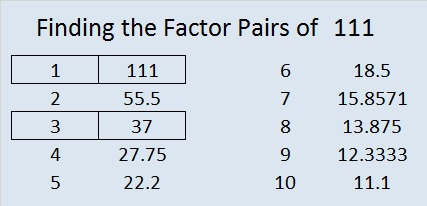111 is never a clue in the FIND THE FACTORS puzzles.

111 = (1 + 2 + 3 + . . . + 35 +36)/6, which can be more easily calculated by using 111 = (36 x 37)/(2 x 6). Therefore, 111 is the magic sum of any 6 x 6 magic square that contains all the integers from 1 to 36.# 23 You Call That a Multiplication Table? THIS is a Multiplication Table

### A Discussion About the Multiplication Table:

Almost everyone is familiar with the traditional multiplication table: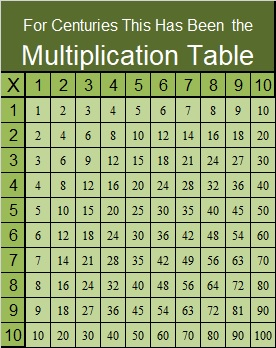Is this traditional way the best way for children to learn multiplication facts? Maybe not!

Beyondtraditionalmath published a post showing the Griddle’s creation of a different kind of multiplication table. This table can really help anyone visualize what multiplication means by relating any multiplication fact to its area. Every child learning to multiply should be exposed to this wonderful table. Even students who already know how to multiply would benefit from looking at it.

On the other hand, I hope it doesn’t completely replace the traditional multiplication table because that old, familiar table is the basis for my Find the Factor puzzles.

### Factors of the Number 23:

• 23 is a prime number.
• Prime factorization: 23 is prime.
• The exponent of prime number 23 is 1. Adding 1 to that exponent we get (1 + 1) = 2. Therefore 23 has exactly 2 factors.
• Factors of 23: 1, 23
• Factor pairs: 23 = 1 x 23
• 23 has no square factors that allow its square root to be simplified. √23 ≈ 4.79583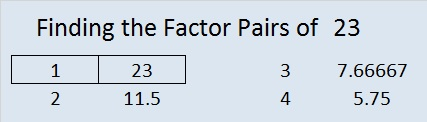How do we know that 23 is a prime number? If 23 were not a prime number, then it would be divisible by at least one prime number less than or equal to √23 ≈ 4.8. Since 23 cannot be divided evenly by 2 or 3, we know that 23 is a prime number.

23 is never a clue in the FIND THE FACTORS puzzles.

### More About the Number 23:

23 is the sum of three consecutive prime numbers:
5 + 7 + 11 = 23.

# 4 Rhyme and Rhythm

4  is the first composite number, and it is 2 squared. 4 = 1 x 4 or 2 x 2. Factors of 4: 1, 2, 4. Prime factorization: 4 = 2 x 2, which can also be written 4 = 2².

Since √4 = 2, a whole number, 4 is a perfect square.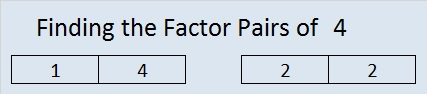When 4 is a clue in the FIND THE FACTORS puzzles, the factors might be 1 and 4 or they might be 2 and 2.

—————————————————————–

Seventeen years ago, when my daughter was learning the multiplication facts, I came across a rhyme that taught one fact:

5, 6, 7, 8……….56 is 7 times 8.

Coincidentally,

1, 2, 3, 4……….I know twelve is 3 times 4.

3 x 4 = 12 isn’t as difficult to remember as 7 x 8 = 56. Still I find it fun to notice the relationship between counting to eight and these two multiplication facts. I enjoyed the rhymes mentioned in my 10/31/2013 post, and I have found yet another site with rhymes for learning the multiplication facts. Two rhymes similar to these 12 and 56 counting rhymes were even included!  The site is : http://www.teacherweb.com/NY/Quogue/MrsLevy/MULTIPLICATION-RHYMES.pdf

Some children have no problem memorizing number facts, but for some children, a rhyme makes learning the facts more fun and much easier.  Even though I already know all of the basic multiplication facts, I am going to memorize these rhymes simply because I personally enjoy them.  I also know I will have at least one opportunity every week to share them with someone trying to memorize the facts: Already when a student asks me, “what’s 7 x 8?” I always answer in rhyme.

Besides the two rhymes listed above, my favorites are:

 Times One: Mirror, mirror look and see, it’s the other number, not me. Times Zero:  Zero is always the hero Six times six / Magic tricks / Abracadabra / thirty-six A tree on skates fell on the floor / Three times eight is twenty-four. A 4 by 4 is a big machine, Iʼm going to get one when Iʼm 16.

This week I even wrote one myself:  Twelve times twelve / Is a dozen dozen / A gross one forty-four / Just ask my cousin.

When a very young child loves a rhyme, he or she will want to hear it over and over again. Mother Goose rhymes have been enriching lives for years. Being able to fully comprehend what the rhyme is about isn’t necessary at first so even preschoolers can be introduced to these rhymes.

Robin Liner writes a blog (crazygoodreaders.wordpress.com) that discusses reading and dyslexia.  On October 5, 2013 , she wrote Rhythm and Rhyme: A Phonological Power Tool.  She wrote, “Rhymes provide subconscious clues.” That means someone is more likely to get an answer right when that answer rhymes with the question. What a fun and powerful way to learn!

Much of what she wrote not only applies to learning to read but also to learning math, science, history, ……. anything.

Twice a week I put 6 new puzzles in an excel file that is attached to this blog. The puzzles can be solved using logic and knowledge of the multiplication table. Here is one of the puzzles I created this week.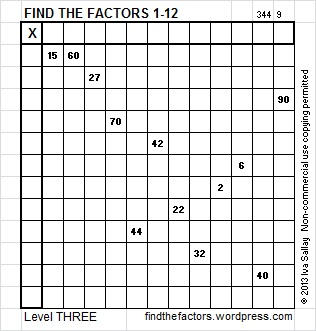Click on this link, 12 Factors 2013-11-07,  for more puzzles and the previous week’s solutions. How do you solve the puzzles? Place the numbers 1 – 12 in both the top row and the first column so that those numbers are the factors of the given clues.

Update: I like the idea of using rhymes to learn multiplication facts so much that I compiled my own list: